Custom SearchREDUCING FRACTIONS TO LOWEST TERMS  There are many useful applications of factoring. One of the most important is that of simplifying algebraic fractions. Fractions that contain algebraic expressions in the numerator or denominator, or both, can be reduced to lower terms, if there are factors common to numerator and denominator. If the terms of a fraction are monomials, common factors are immediately apparent, as in the following expression: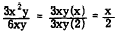If the terms of a fraction are polynomials, the polynomials must be factored in order to recognize the existence of common factors, as in the following two examples: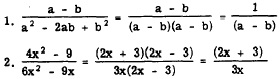Notice that without the valuable process of factoring, we would be forced to use the fractions in their more complicated form. When there are factors common to both numerator and denominator, it is obviously more practical to cancel them (first using the factoring process) before proceeding. Practice problems. Reduce to lowest terms in each of the following: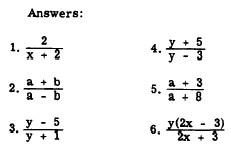OPERATIONS INVOLVING FRACTIONS  Addition, subtraction, multiplication, and division operations involving algebraic fractions are often simplified by means of factoring, whereas they would be quite complicated with- out the use of factoring. MULTIPLYING FRACTIONS Multiplication of fractions that contain polynomials is similar to multiplication of fractions that contain only arithmetic numbers. If this fact is kept in mind, the student will have little difficulty in mastering multiplication in algebra. For instance, we recall that to multiply a fraction by a whole number, we simply multiply the numerator by the whole number. This ,is illustrated in the following example: Arithmetic: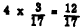Algebra: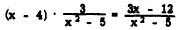Sometimes the work may be simplified by factoring and canceling before carrying out this multiplication.  The following example illustrates this: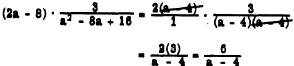When the multiplier is a fraction, the ruler of arithmetic remain applicable-that is, multiply numerators together and denominators together, This is illustrated ,as follows: Arithmetic: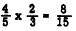Algebra: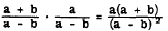Where possible, the work may be considerably reduced by factoring, canceling, and then carrying out the multiplication, as in the following example:Although the factors may be multiplied to form two trinomials as shown, it is usually sufficient to leave the answer in factored form. Practice problems. In the following problems, multiply as indicated: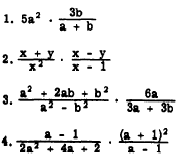Answers: